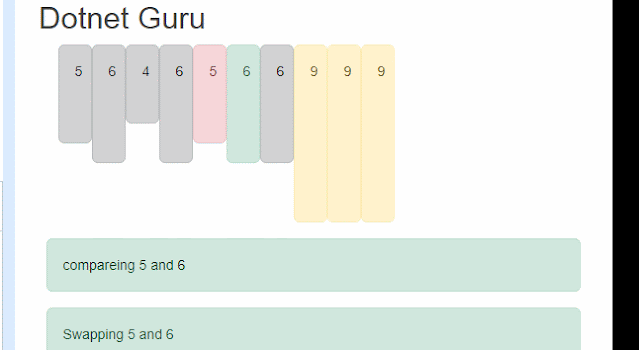# How to Visualize sorting algorithm using Blazor

0

Microsoft’s Blazor is a cutting-edge technology for creating applications that run on the web. Blazor supports both a server-based and a client-based rendering model. In this article, I will demonstrate how to animate a sorting algorithm, precisely bubble sort so that you can use it in your projects.

I will assume that we are familiar with the Blazor application before we move on to implementing the sorting.``````<div class="container">
<div class="d-flex flex-row">
@for (int i = 0; i < @Items.Length; i++)
{

@if(Items[i].IsSorted==false){
<div class="@Items[i].CssClass"  style="height:@(Items[i].Value*20)px;width:10px">@Items[i].Value</div>
}else{
}

}
</div>
<div class="row">

@if (swapped)
{
}else{
}
</div>
</div>
@code{

private string swapText = "";
private string compareText = "";
private Item[]? Items { get;set;}
private Random rand = new Random();
private bool swapped = false;
protected override void OnInitialized()
{
Items = Enumerable.Range(1, 10).Select((v, i) => new Item()
{

Value = rand.Next(1, 10)

}).ToArray();

Sort();
}

{

for (int j = 0; j < Items.Length; j++)
{

for (int i = 0; i < Items.Length - 1; i++)
{

compareText = \$"compareing  {Items[i + 1].Value} and {Items[i].Value} ";
if (Items[i].Value > Items[i + 1].Value)
{

swapText = \$"Swapping   {Items[i + 1].Value} and {Items[i].Value}";
(Items[i].Value, Items[i + 1].Value) = (Items[i + 1].Value, Items[i].Value);
swapped = true;
StateHasChanged();
swapText = "";
}
compareText = "";
swapped = false;
}
Items[Items.Length - j - 1].IsSorted = true;
StateHasChanged();
}

}
}
``````

Let’s understand the code. The main logic is inside the `Sort` method. There is nothing special about the code. It’s a simple c# code for sorting the array. The only tricky thing is updating the CSS class before comparing the array item and then updating the CSS class when there is a swap. Now the role of blazor is involved when we are changing the CSS class; we are forcing blazor to re-render the UI by calling the `StateHasChanged` method.
Lastly, I am updating the index of the previous item to sorted and updating the CSS class to the final state.

In the code, I am using one Item class shown below and one extension method to update the CSS class of the corresponding array item.

Item.cs

``````  public class Item
{ public bool IsSorted { get; set; } = false;
public int Value { get; set; }
}
``````

CssExtensions.cs

``````  public static class CssExtensions
{
public static void UpdateClass(this Item[] items, int index, string className)
{
items[index].CssClass = className;
}
}

``````

Tags